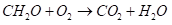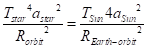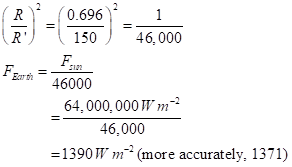# test MT to image

Converting MathType equations to images:

Kutools converted very few equations to images!

Saving a docx as html had the same problem.

Then I simply copied any MT equation, pasted it in a docx like this one, and pasted it in with the 4th choice, as image!

The choice icon looks like a mountain scene!

Now check that this conversion to image does work. Put this document onto a webpage with Mammoth .docx conveter1. (in free space). Then

This is classic one-over-r-squared law for the falloff of power with distance. With R = radius of the Sun (0.696 million km) and R’ = mean radius of the Earth’s orbit (150 million km),If we want a planet with an energy flux density that’s the same as for Earth (so that it has about the same temperature), we want the total power of the star spread out at the planet’s orbital distance to be like that for the Earth: